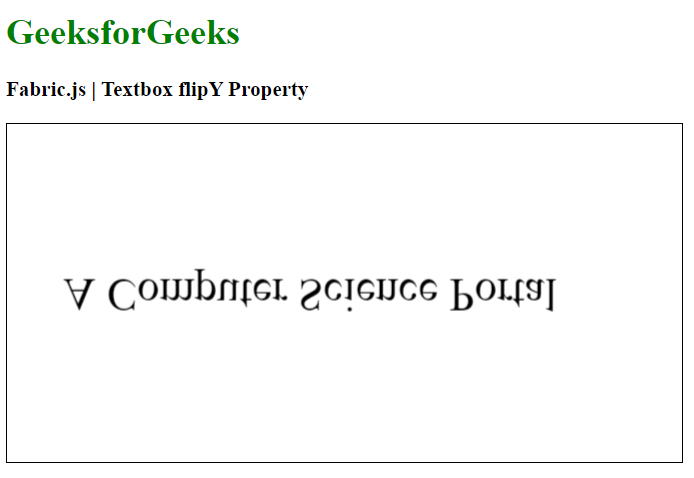Related Articles
Fabric.js Textbox flipY Property
• Last Updated : 19 Jan, 2021

In this article, we are going to see how to vertically flip a Textbox canvas using FabricJS. The canvas Textbox means Textbox is movable and can be stretched according to requirement. Further, the Textbox can be customized when it comes to initial stroke color, height, width, fill color, or stroke width.

Approach:

• To make it possible, we are going to use a JavaScript library called FabricJS.
• After importing the library, we will create a canvas block in the body tag which will contain the Textbox.
• After this, we will initialize instances of Canvas Textbox provided by FabricJS and set the flipY property and render the Textbox on the Canvas as given in the below example.

Syntax:

```fabric.Textbox('text', {
flipY: boolean
});```

Parameters: This function accepts one parameter as mentioned above and described below:

• flipY: It specifies that the object flip vertically .

Example: This example uses FabricJS to flip the canvas Textbox vertically.

## HTML

 ```<``html``>`` ` `<``head``>``    ``<``title``>``        ``Fabric.js | Textbox flipY Property``    ```` ` `    ````    ``<``script` `src``=``"https://cdnjs.cloudflare.com/ajax/libs/fabric.js/3.6.2/fabric.min.js"``>``    `````` ` `<``body``>``    ``<``h1` `style``=``"color: green;"``>``        ``GeeksforGeeks``    ```` ` `    ``<``h3``>``        ``Fabric.js | Textbox flipY Property``    ```` ` `    ``<``canvas` `id``=``"canvas"` `width``=``"600"` `height``=``"300"` `        ``style``=``"border:1px solid #000000"``>``    ```` ` `    ``<``script``>`` ` `        ``// Initiate a Canvas instance ``        ``var canvas = new fabric.Canvas("canvas");`` ` `        ``// Create a new Textbox instance ``        ``var text = new fabric.Textbox(``            ``'A Computer Science Portal', {``            ``width: 500,``            ``flipY: true``        ``});`` ` `        ``// Render the Textbox in canvas ``        ``canvas.add(text);``        ``canvas.centerObject(text);``    `````` ` ``

Output:My Personal Notes arrow_drop_up